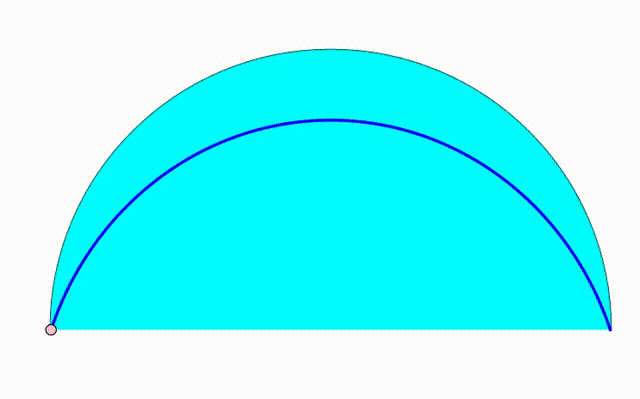# Dynamic Geometry: P90

Geometry Level pendingThe diagram shows a black semicircle. The cyan and green semicircle are growing and shrinking freely on the black semicirle's diameter. The purple circle is internally tangent to the black semicircle and tangent to both green and cyan semicircle. The red and orange circles are internally tangent to the black semicircle and tangent to the purple circle and to one of the bottom semicircles. The center of the orange and red circles trace a locus (blue curve). The area bounded by the blue curve and the black semicircle's diameter can be expressed as:

$\:\:\frac{p}{q^5}\left(p^2\sqrt{q}\sin ^{-1}\left(\frac{q\sqrt{q}}{p}\right)-q^2\right)$

where $p$ and $q$ are coprime positive integers. Find $p+q$.

×